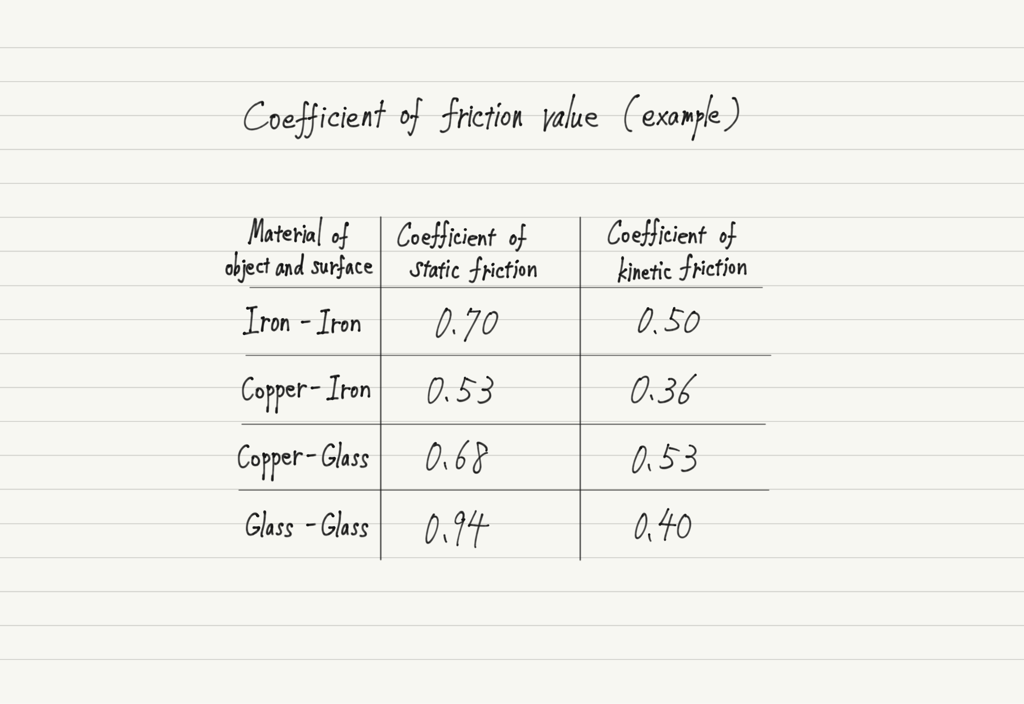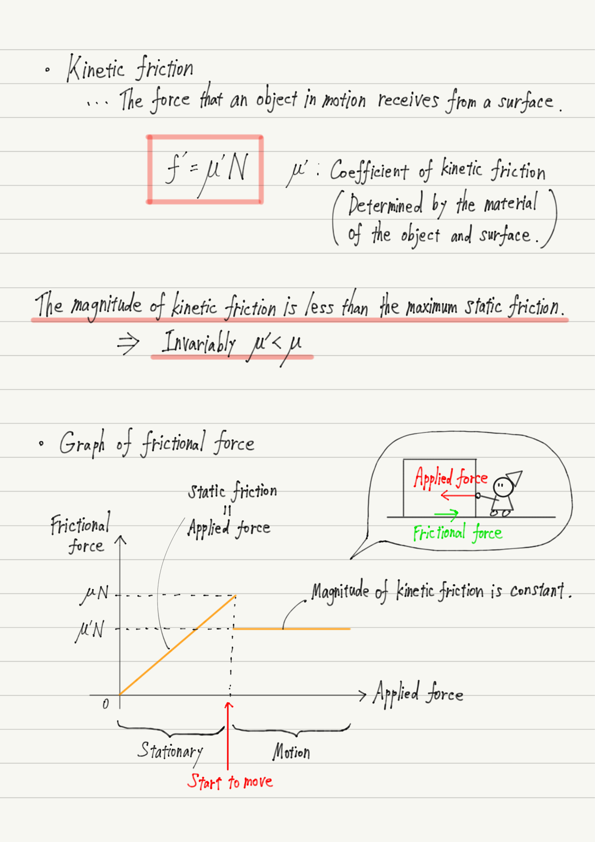# Kinetic Friction

Continuing from the previous lecture, this time we will again deal with frictional forces. First, let’s review the static friction we studied last time.

When a force is applied to move an object, if the applied force is small, the object will not start moving.
This is because a static friction of the same magnitude as the applied force is generated.

However, there is a limit to the magnitude of the static friction (Maximum static friction), so increasing the applied force causes the object to move.
When the magnitude of the applied force equals the maximum static friction, that is the moment when the object starts to move!

This is all we have talked about so far in the previous lecture.
This time, let’s learn about the frictional force after it starts moving!

## Frictional force when an object is moving

When the applied force exceeds the maximum static friction, the object starts to move, but the frictional force has not disappeared.
It has not disappeared, but the type of frictional force has changed.
Until the object begins to move, a static friction is acting on it, but after it begins to move, the frictional force changes to a kinetic friction.

Kinetic friction is a force that acts in the direction that interferes with movement.
This is similar to your image of frictional force, isn’t it?

Now, the magnitude of the kinetic friction f’ can be calculated as well as the maximum static friction.
The formula is f’=μ’N.
Very similar to the formula fN for finding the magnitude of the maximum static friction f !
Note that the only difference is in the proportionality constant.

## Relationship between the two coefficients of friction

μ’ is called the coefficient of kinetic friction and, like the static coefficient of static friction μ, is determined by the material of the object and the surface.
Here are some examples.Notice the relationship between the static and kinetic coefficients of friction in the table above.
All of them have μ>μ’ !

This is no coincidence; for all materials, the coefficient of kinetic friction is always smaller than the coefficient of static friction.

When you push a heavy object to move it, it is difficult until it starts moving, but once it starts moving, the rest is easy. Have you ever had such an experience?
This is because the coefficient of kinetic friction is smaller than the coefficient of static friction.

Finally, it is clear from the formula that the magnitude of the kinetic friction remains constant while the object is in motion!
This is a major difference from the static friction, which changes its magnitude depending on the force applied.

## Summary of this lecture## 次回予告

This is the end of a series of articles introducing various types of force.
In the next lecture, we will deal with force calculations.

https://www.yukimura-physics.com/entry/dyn-f17

error: Content is protected !!